## Subscribe to our mailing list

* indicates required

Audit !!!

Check out our RefTreeAnalyser
the ultimate Excel formula auditing tool.

Trainings

Excel VBA Masterclass (English)
Excel VBA for Financials (Dutch)

Third party tools

FastExcel
The best tool to optimise your Excel model!

Stellar Phoenix Excel Repair
Best tool to repair corrupt Excel sheets and objects
Home > English site > Articles > Transpose Table# Transposing the Formulas in a Table of Cells

Recently someone asked me if it was possible to transpose a table of cells, but in such a way that the formula of each cell is kept intact. I decided VBA was the way to go and produced a small but very useful little routine for that.

Imagine the following table: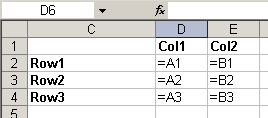Now I'd like to transform that table into this one (see highlighted range):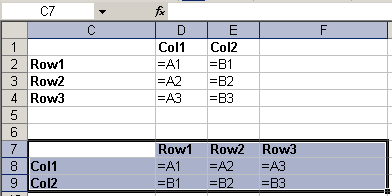If you try a copy > paste special > formulas > transpose, this is what you get: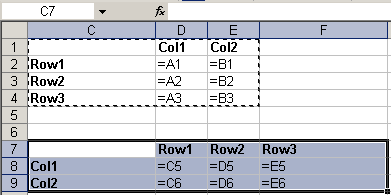But it is not what we want, is it.

With the following piece of VBA, you can easily transpose your table, without garbling your formulas:

Option Explicit

Sub TransposeFormulas()
Dim vFormulas As Variant
Dim oSel As Range
If TypeName(Selection) <> "Range" Then
MsgBox "Please select a range of cells first.", _
vbOKOnly + vbInformation, "Transpose formulas"
Exit Sub
End If
Set oSel = Selection
vFormulas = oSel.Formula
vFormulas = Application.WorksheetFunction.Transpose(vFormulas)
oSel.Offset(oSel.Rows.Count + 2).Resize(oSel.Columns.Count, oSel.Rows.Count).Formula = vFormulas
End Sub

So how does this work?

First we check whether a range of cells has been selected, otherwise the code will produce a runtime error:

If TypeName(Selection) <> "Range" Then
MsgBox "Please select a range of cells first.", _
vbOKOnly + vbInformation, "Transpose formulas"
Exit Sub
End If

Next, we store the selected cells in an object variable (which is not really necessary), so we can work with that set of cells:

Set oSel = Selection

Now the formulas of these cells are pulled into a variable of type Variant in one go:

vFormulas = oSel.Formula

If you step through the code (using my example) the Locals window of the Visual Basic Editor (VBE) shows this for the variable vFormulas after executing the statement above: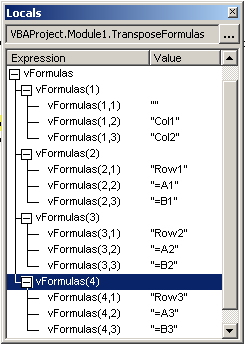As you can see, vFormulas is a 4*3 array.

Next we want to swap rows and columns of the vFormulas variable, for which I use the Transpose worksheet function:

vFormulas = Application.WorksheetFunction.Transpose(vFormulas)

The structure of vFormulas changes to this: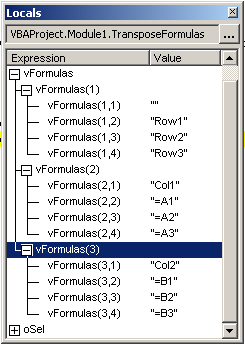Presto! We now have a 4 * 3 array.

Finally, we need to push back the formulas to the worksheet. To be able to do that, we must specify a target range of the exact dimensions as the array variable. For that I use the Resize method. Note I also move down a couple of cells using Offset, so the copy of the table is placed 2 rows below the original:

oSel.Offset(oSel.Rows.Count + 2).Resize(oSel.Columns.Count, oSel.Rows.Count).Formula = vFormulas

Done!

Comment by: michael fozouni (6-3-2013 04:31:58)

Hi,
First, let me extend my sincere thanks and gratitude for all your work in providing great tips on excel.

Second, I ran your "Transpose" macro without selecting a range first. However, the routine did not prompt to ask me to select a range. Am I doing something wrong here maybe?

Michael

Comment by: Jan Karel Pieterse (6-3-2013 11:35:25)

Hi Michael,

The macro takes whatever the current selection is and pastes the transposed formulas of that selection below the selection. There are no prompts.

Comment by: Michael (6-3-2013 13:01:04)

Dear Jan,
Thanks much for your prompt response. I guess whereI fail to understand is the message box where it asks you to select a range?

In any event, many many thanks for answering my question and once again, congratulations on your invaluable site!

Michael

Comment by: Jan Karel Pieterse (6-3-2013 19:34:25)

The message box should only pop up when you have something else selected, like a chart.

Comment by: Michael (6-3-2013 20:12:31)

Got it, thanks.
Michael

Comment by: luciana (26-9-2013 20:19:11)

Perfect VBA!! Helped a lot!! Only one question... Is there a way to keep the formating from the orginal table? because when I use the simple Â´transposeÂ´ from excel it keeps my formating, like writing in different color, or a cell painted in another color, etc, but it messes up the formulas. but if I use this Macro it keeps the formulas perfectly, but I would have to format all over again.... is there a way to insert the formating in the VBA? I think there isnÂ´t, just thought I should confirm first.
Thank you very much for the Macro though... helps a lot!

Comment by: Jan Karel Pieterse (27-9-2013 11:17:58)

Hi Luciana,

You could add the paste-special transpose to the macro as a first step, after which you let the rest of the macro do the formula trick:

Sub TransposeFormulas()
Dim vFormulas As Variant
Dim oSel As Range
If TypeName(Selection) <> "Range" Then
MsgBox "Please select a range of cells first.", _
vbOKOnly + vbInformation, "Transpose formulas"
Exit Sub
End If
Set oSel = Selection
vFormulas = oSel.Formula
vFormulas = Application.WorksheetFunction.Transpose(vFormulas)
oSel.Copy
oSel.Offset(oSel.Rows.Count + 2).PasteSpecial Paste:=xlPasteAll, Transpose:=True
oSel.Offset(oSel.Rows.Count + 2).Resize(oSel.Columns.Count, oSel.Rows.Count).Formula = vFormulas
End Sub

Comment by: luciana (27-9-2013 20:35:01)

It worked perfectly! Thank you!

### Have a question, comment or suggestion? Then please use this form.

If your question is not directly related to this web page, but rather a more general "How do I do this" Excel question, then I advise you to ask your question here: www.eileenslounge.com.